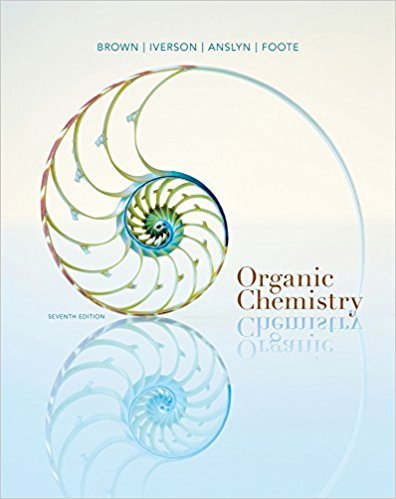×

×

# Solutions for Chapter 17: Carboxylic Acids## Full solutions for Organic Chemistry | 7th Edition

ISBN: 9781133952848Solutions for Chapter 17: Carboxylic Acids

Solutions for Chapter 17
4 5 0 431 Reviews
17
1
##### ISBN: 9781133952848

This textbook survival guide was created for the textbook: Organic Chemistry, edition: 7. Since 48 problems in chapter 17: Carboxylic Acids have been answered, more than 56737 students have viewed full step-by-step solutions from this chapter. Chapter 17: Carboxylic Acids includes 48 full step-by-step solutions. This expansive textbook survival guide covers the following chapters and their solutions. Organic Chemistry was written by and is associated to the ISBN: 9781133952848.

Key Chemistry Terms and definitions covered in this textbook
• alkynes

Hydrocarbons containing one or more carbon–carbon triple bonds. (Section 24.2)

• Aromatic compound

A term used initially to classify benzene and its derivatives. More accurately, it is used to classify any compound that meets the Hückel criteria for aromaticity (Section 21.2A).

• calorimetry

The experimental measurement of heat produced in chemical and physical processes. (Section 5.5)

• chlorohydrin

A compound containing a Cl group and a hydroxyl group (OH) on adjacent carbon atoms.

• Dehydration

Elimination of water.

• diamagnetic anisotropy

An effect that causes different regions of space to be characterized by different magnetic field strengths.

• Graham’s law

A law stating that the rate of effusion of a gas is inversely proportional to the square root of its molecular weight. (Section 10.8)

• Hybrid orbital

An orbital formed by the combination of two or more atomic orbitals.

• hydrogen deficiency index (HDI)

A measure of the number of degrees of unsaturation in a compound.

• Hückel’s rule

The requirement for an odd number of p electron pairs in order for a compound to be aromatic.

• monomers

Molecules with low molecular weights, which can be joined together (polymerized) to form a polymer. (Section 12.8)

• Nitrogen rule

A rule stating that the molecular ion of a compound with an odd number of nitrogen atoms has an odd m/z ratio; if zero or an even number of nitrogen atoms, the molecular ion has an even m/z ratio

• Nucleophilicity

A kinetic property measured by the rate at which a nucleophile causes nucleophilic substitution on a reference compound under a standardized set of experimental conditions.

• overall reaction order

The sum of the reaction orders of all the reactants appearing in the rate expression when the rate can be expressed as rate = k3A4a 3B4b... . (Section 14.3)

• Plastic

A polymer that can be molded when hot and retains its shape when cooled

• reaction rate

A measure of the decrease in concentration of a reactant or the increase in concentration of a product with time. (Section 14.2)

• Reduction

The gain of electrons. Alternatively, either the gain of hydrogen, loss of oxygen, or both

• retrosynthetic analysis

A systematic set of principles that enable the design of a synthetic route by working backward from the desired product.

• saturated hydrocarbon

A hydrocarbon that contains no p bonds.

• tosylate

An excellent leaving group (OTs). transition state (Sect. 6.6): A state through which a reaction passes. On an energy diagram, a transition state corresponds with a local maximum.